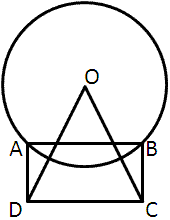# Knewton Brutal Challenge - Week 13 (Math Edition)

by , Jun 23, 2010

There's only one way to get a Knewton t-shirt free today. And that is to answer this question correctly and show your work.

In the below diagram, points A and B lie on the circle with center O, and rectangle ABCD and triangle ODC have the same area. If the area of the circle with center O is [pmath]pi[/pmath] times the area of rectangle ABCD, what is the ratio of the length of the radius of the circle to the length of segment DC?

(A) [pmath]2 : 1[/pmath]

(B) [pmath]2 : sqrt{2}[/pmath]

(C) [pmath]1 : 1[/pmath]

(D) [pmath]1 : sqrt{2}[/pmath]

(E) [pmath]1 : 2[/pmath]